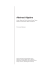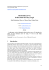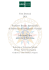# Epsilon Nets and

## Transcription

Epsilon Nets and
15
EpsilonNetsand
Tlansversalsof Hypergraphs
More
For historicalreasons,
a finiteset-system
is oftencalleda hypergraph.
prccisely,
^ htperqraph
H consists
of a finitesety(il) of terticer(points)and
of E(fl) are usuallycalled
a family E(fl) of subsets
of y(H). The elements
(or, ft short,edgex).If the hyperedges
of I' arer-elementsets,then
hyperedges
l, is saidto be an /-rnrlormhlperyraph.Usingthisterminology,
a graphis a
two-uniform
hypergraph.
In Chapterl0 wehaveextended
somegraph-theoretic
(cf. Theorems
resultsto r-uniformhypergraphs
l0.ll and 10.12).
The conceptof hypergraphs
is a very generalone,so it is not surprising
in variouslieldsof
thathypergraph
theoryhasa largescaleof applications
l', a subset
I g y(fl) is
mathematics,
includinggeometry.
Civ€na hypergraph
for everyedgeE € E(H).Many
calleda tra s|e$al of H if ?n E is nonempty andgeometry canbe reformulated as extremalproblemsfrom combinatorics queslions in a lransversal of thefollowingtype:Whatis thesizeof a smallesl givenhypergraph H? Thisproblem, in general, is knownto becomputationally (cf. Careyand Johnson,1979).However,undercertainspecific intractable conditionson ll, one can guarantee the existenceof a relativelysmall transversal. The presentchapterfbcuseson resulhof this kind.In particular, we shallseehow a powerfulprobabilistic ideaof Vapnikandchervonenkis geometric and algorithmic canbe appliedto obtaina numberof interesting results, TRANSVERSALSAND FRACTIONAL TRANSVERSALS t€t fl bea hypergraph withvercx sety(It) andedgeset,.(H).Letr(1{)denote thesizeof a smallest transversal of fl, thatis, thesmallest number7 suchthat onecanchoose vertices withthepropenythatanyedgeofli contains at least " is usuallycalledtherrdnrlendlnumber(ot thewrlet-cover oneofthem.7(I1) umber)of H. Thepackingnumber(ot matchinq number\of ahypergraph l/ is definedas thelargestnumber/ = /(li) suchthatfl has, pairwisedisjointhyperedges. Obviously, /(l1) < r(a) for anyhypergraph H. Typically, 7(li) is strictlylarger a Epsilon Neti and TllNversrls of Hyperyraphs + rt t a a + .i a a than /(fl). In fact, 7(H) cannotevenbe boundedby any functionof I,(H) (see Exercise15.3). Let lll" denotethe setofall nonnegative real numbers.l-et us call a function l: y(A) -+ lR' a fractioaal transyersal of H if (.r) > | (15.t) Sin The minimumof >€v(fl) l(r) over all fractionaltransversals of ft is calledthr tactional transyersalnumberof H , andis denotedby r* (fl). Onecanassociate with eachtransversal? of ll a functiont.: y(H) -+ ll{+ definedas Ihu ) ",',-{l l[ili: * - rD . t .D ID lbr evervhvperedsef € E(H). (15.1)and L. v(ol7(,r) = lll, we havethar Sincethis functionsatisnes t- (H\ <r(H). Similarly, afractionalpactrnSof Il is a nonnegative functionp: E(H) -+ llt' suchthat [email protected]\<l for everyvertexr c Y(i1). ihe -'lu :e\ <D t (- tC .- The maximumof >.e.(fi )p(E) over all fractionalpackingsof 11 is calledthe fuactiokolpackingnunber of 11,and is denotedby /"(H). As before,we hale v'(H) > v(H). It is easy to deducedirectly from the definitionthat /'(fl) < i'(H) (see Exercisel5.l). In fact, thesetwo numbersare always equal to each other. Moreover,the following is true. it Th€orem 15.1. For e,reryhtpergraphH, t rt - -a -- v(H) < Y'(H, = r' (H) < 'r(H), l :,n l,'f Th€ P and the valueof r'(H\ = r'(H) can be determinedby linear pmgrammiftg -a -+ -t -'a + Proof, Let,ri (1 < i < r,) and tJ (1 <j < ,.) be the verticesand rhe edges of 11,respectively. Let A = (aij\ be the incidencemattix of H, i.e., = | 1 if rieEj, ",i lo r ,,erj. Let A7 denotethe transpose of A, and let 1,,denotethe matrixconsisiingof one columnof lengthr, all of whoseentriesare 1's.civen a functionr: y(H) ) li (^nd p: E(H, + lli), let I (rcsp.p) denotea matrix consistingof one column whoseith entry is r(ri) (resp.p(E-)). ..n and T.amvebrlsof flJp€rgnphs by anyfunctionof /(fl) (see I}msv€ruols and lrrctiond T.lDsveBlls Observethat t is a fractionaltransversalof 1{ if and only if rmbers. Let uscalla function CeE e E(Hr. ( l s .)r ranxversals ofH is calledthe lby r'(lt). Ooecanassociate -+ Il* definedas ,ttlx) = lTl, w€ have rhal ativefunctionprE(/t) + ill' ( ir € Y(It). I packingsof fl is calledthc r, ,'(l/). As before,we have )n that r'(ft < r'(I/) (see rlways equal to each other 245 A7l>1,, and !>0. Similarly,p is a fracrionalpackingof 11if andonly if Ap<1,, ^nd p>O, Thus, r'(I1) = min{llllArl > 1,,,! > 0}, v'@)=n x {rIp_lAp< thp>O}. problems Thesetwo linearprogramming aredualto eachother,so it follows immediately from the duality theorem of linear prograrnmingthat their solutions, r'(f4 and r'(tl), areequal(see,e.9.,Papadimitriou and Steiglitz, 1982;Chvital,1983;andCrijtschelet al., 1987). n In general, r'(l/) canbe muchsmallerthan7(fl) (seeExercise15.3).The followingtheorem of Lov6sz(1975)showslhatlhisis ooithecasewhenevery pointof lJ belongsto relativelyfew hyperedg€s, Theorem 15,2 (InvAsz), kt H be a hypergraphwhoseerery ve ex is conlainedin at fiost D edges.Then -l 7'(lt) < r(H) < (ln D+ I)r'(fl). H), by linear Wogmmming. , the vertices and the edges Proof. We haveto proveonly the secondinequality.Let t: y(fl) + R+ be a fractional transversal of fl with L€vrr) (.r) = r'(fi). We arc goingto selecta setof venices.rt,xz,...by a greedyalgoithm. Let xr b€any ve(exof H whosedegree(i.e.,thenumberof edgescontaining it) is maximal,Let Dt denotethe degreeofxr in lt. Setllr = FI .rt, thal is, the hypergraph obtainedfrom H by deletingth€ vertex.rt andall €dges containing.rt.If-r1,...,ii e y(A) havealr€ady beenselected, thenlet Ilr = H xt - t2 - ... - ri. lf Hi hasno edges,we stop.Otherwis€, let ri*r be a vertexof l/i whosedegreeDr*t is maximal,andso on.Clearly, h€ matrix consisting of one en a function.: Y(It) ) lll consistingof one column lE(H)l - lE(Hi. t)l= Di.t. By theproperties of ,, (15.2\ aa .l -a 2$
F4sllon Neb.nd ftrl|lveNk
ol Hyp.rgnpls
lE(ri)l=
> r<Ee>gHl
Ee ElHt)
=I<'rI
r<YlHl
< )
I
Ee E(Hl
Etr
(')D,-'
3 Di+tt'(Hr.
Assumenowthatour proccdureterminaiesin s steps;i.e.,t , is empty.Then.
of course,
r(H) < r. PutII0 = H. By (15.2),we h.ve
,= -l,/ L / n = S lE(t/)l-lE(I1ar)l
r
tE#r)l
_ ___F_
=
+,\-,.,-,,r
).p\nt1ll'\
'!u'
ot
D*r
_r\
Dt l'
Hence,usingtheinequality
lE(A)l < Di+ri"(fl) (0 < j < s) andthefactD" > l.
we obtain
" <"1a1*!a,-,"'{r,(E;i;)
= , ' '1\ a
s y+*1D!1, J D , a * ' 1
.r,u,('-;-=t.,
+)
=",r"r(r._j,f;
< r'(H)(l + ln Dr).
Thus,
7(fl) <s <7'(g)(l + ln Dr),
as desired.
siton Netsmd lianNe6ds of H}lergraphj
<s
r(j)
.,s
I
r )Di+|
L
inatesin r stepsii.e.,iI* is empty.Then,
i.2), we have
,, )l lE(H,+r)l
D*t
,
I
I\
'' \ o*,='
a)
.r7 (H) (0 < i < s) andthefactDr > I,
.
;rnt\
I
^
,
,, D,+r\
DI
tlr
t\
r)
t\
g)
Vapnil-Che.voneDl*
u7
DimeBlon
VAPNIK-CIIERVONENKTS DIMENSION
Suppose
that for a publicopinionpolt we wantto selecla smallnumberof
individualsrepresenting
all majorsectionsof the society.First,we haveto
choosecerlain categoriesof fEople and then decidewhich of thesegroups
arc considered"importaot."Accordingto our democraticprinciples,we sh;lt
measure
the "importance"
of a groupby its size(in the percentage
of the
population).
Thenthe importantgroupswill definea hypergraph
fl with the
propenythatlEl > €ly(fl)l for everyedg€E € E(li), where€ is someflxed
(0 < € < l). Thesmallest
conslant
numberof peoplerepresenting
all important
groupsis r(fl).
Clearly,th€ functiont(xt = l/(elv(H)l'r,for att, € y(H), is a fractional
traNversalof F/ with >.vott(x) = l/e. Hence,r'(fl) < l/e, andTheorcm
15,2imDliesthat
r1a;<11tna+11,
(rs.3)
where, is themaximumdegree
of thevertices
of It. Thisboundis extremely
poorif D is larye.
In theirseminalpaper,VapnikandChervonenkis
(1971)pointedout thatif
It satisnescertainoaturalconditions,the aboveupperboundcan be replaced
by a functiondepending
onlyon €. To specifytheseconditions,
we need_
some
pfepamtron.
Defnitiarn 15.3. Let H = (V(H),E(H, denote a hyp€rgraph.A subset
4 E y(tf) is caffedshattererl
if for everyB C ,4 thereexistsan E € E(Il)
suchthat En A = 8. Th. Vapnik-Chenonenkis
dintension(or VC dime sion\
shattered
subser
of V(H).Il will bedenorcd
by vc-dim(Ii).
'Thefollowing
theorcmwasprovedindependently
by Shelah(19?2),Sauer
(1972),andVapnikandCh€rvonenkis
0971).
Theorem15.4. Let H be a hyperqtupllwith n yertice!and Vc-dimensiond.
The
l r t s r l s/, ,1r\,+i,/,1, \+ * ( a l
and this bound ca not be improved.
)(l+lnDr),
First Proof, The assertionis tdvial ifd = 0 or', < d. Assumethatwe have
akeadyprovedil for every hypergraphF wilh Vc-dim (H-r< d. and lbr every
-la anO
hypergraph
H wirhvC-a;mrlir
lytntl< z.
Givena hypergraph
H with', vertices
andVc-dimension
d, letusdefinetwo
--
I;psilon Nc(g and Tta.sv€rsals ol Hypelgraphi
e-J
otherhyperSraphs,
llr and H2, as fbllows. Let V(H t) = V(H) = V(H)
for somenxed .r e Y(Ii), and set
a-
Irl
E(Ht)= lE UllE e E(H\\,
E ( H ) = l E e E ( H ) l rd E a n d E U h l e E ( H ) ) .
-l-
--
rD_
.D
-)
.-
Obviously,
Vc-dim(Hr)<dandVc-dim(dz)<l
l.
On theolherhand.by rheInduct,on
hyporhesis.
lE(H)l= |E(Ht)l+ lE(Hr)
st/".'\*!/".'\
!,r\tt4\tl
<
a-
=t/'r\
-
.fa
a-
i)
.l-
-a
t-
tID
'Ir
-t
..-
The tighlness of tni* mun,t follows from the lrcr that il ,(H)
(rr)=/.
vc-dim
{Uc YI lUl< /}, then
We also includea slightly more complicaledproof due to Frankl and P!!h
(1983),becNsc it is a good illustrationof the so-c led line$altehru netht\; (see,e.9.,Bab.i AndFrankl, 1988). SecondProof. Let E(fl) = {E,l I < i <,r}, and let Xr. | < j < >11,,(1 t. b" a list of all subsetsof y(lt) of size ar most d. Definean ,, x >'/.{, ('jJ marn\ 6 = (a,) b] Il ,r=10 iff,rx. if E, b x,. Suppose,for contradiction,that ,i > Z;=.1;l lhen lhe rowror A .,r.' Iinearly dependentover the reals; thus there existxa nonzerofunclio: /: E(fl) ) .ll suchthat ) rtar=o for everyXr. LEt A e V(H) be a ,ninirnclsubsetfor which - l./ta,t="+0. (SetsA with nonzerosumscenainlyexist, for we get a nonzerosum fbr xnl ma\imal elementA of the family {A e 6(A)l/(4) I 0}.) Obviously,lAl > /+ l Given any B g A, let r and Transveredsof Hyperyraphs vlpnik-CheNonenkis Dimosion v(H)=v(H)=V(H)-I') F(B)= tL / " /(8,). EiaA-B Thus, F(A) = d, and setting I = A - {r} lor any fi xed d e A, EU tile E(I1)]. id is, r,p\- S L rir., Ei2B S r,r\ EiaA =0-a=-a In general, if I is any(lAl- t)-elementsubs€t of A (0 <,t < lA ), rhen /,-l\ F(B)=(-t)td+0. This yields,in particular,thatthereexistsat leastonehypercdge Ei with EinA = B. Thus,A is shatter€d, contradicting our assumption lhatVc-dim(fl) = d. O n the fact that tf E(H) = n proof due to Frankland Pach )-calledlinear algebramethod nd letxj, 1 < j < >':{,(';), be )efineanmx >Lo{'l) matrix (1971)discovered Vapnik and Chervonenkis an ingeniousprobabilistic (counting) argument basedon the abover€sult,whichleadsto a substantial improvement of the bound(15.3),They showed(in a somewhat different setting)that thereexistsa function/(d,€) suchthat the transversal number of everyhypergmph 11of Vc-dimensiond, all of whoseedgeshaveat least €ly(I/)l €lements, is at most/(d, e) (seeExercise15.6),Theideasof Vapnik andChervonenkis havebeenadapted by Haussler andWelzl(1987)andBlumer et al, (1989)to obtainvariousupperboundsod /(d,€). Theseresultswere sharpened (1992),asfollows. andgen€ralized by Koml6s,Pach,andWoeginger Civen a finite s€t y, a functionp: V + ll{+ is c lled a probabilitymeasureif = l. tL . - - at.r) ' :t, l). Th€nthe rows of A are e existsa nonzerofunction Themeasure of anysubset X g y is definedby p(X) = >€ x t(i) Theorem 15.5 (Komf6set al.). Iet H be a hypergraphof Vc-dime siond, let . > 0, and let p be a probabilil! measureon V(H) suchthat p(E) > Efor everyE e E(H).Thenr(H) < t(d,.r, wherct(d,e)denotes positive thesmallest integert satislti g z!/lrir _.J\it\. .1)''-"'.r rt tly,for atryE< i, wehave for someintegerT > t. Conseque we get a nonzero sum for any l) / 0).) Obviously, lAl > d+ 1. ,vttt!(nl+zrnrn 1* o\ €\ € e (cf. Exercise15.9). / 250 EpslloDNeh rnd llansveru€ls ot Hypergrrpht Proof. Let us selectwith possibl€repetitionr mndompointsof y(H), wherethe selectionsare donewith resp€ctto the probabilitymeasurer. We geta rundomsample t e l v ( H ) 1=|v ( H , x xv(H). we saythat r is a traflrveEol ot H if ewry edgeE € E(l/) containsat least onepointof r, Let /(8,.r) denotethenumberof components of r thatbelonS Thcn to E, countingwith multiplicity. Prtr is not a [ansversal of fl] = Pr[3E € E(Hrt I(E,x)=01. Havingpickedthe stringr of lengthl, let us chooserandomlyanotherT I efements from V(H\. Lgt , I IV(H\lr1 denotethis new stdng,and let . Furth€rmore, let (z) = (ry) denotethc .r) e [V(,/)lr standfor the full sequence. occurring in z (i.e.,theyarccounted with multiplicilicr multiretofall elements but theirorderis irrelevant). ForanyE € E(It), l(E,t) is a randomvariable havingbinomialdistdbul;on. Let mEbe the medianof I(E,t , PrII(E,9 > '/l.|l< + <tulI(E,y, > nd. The following inequalityis an immediatecons€quence of the independencc of .t and,. Pr[3 E € E(r4 r1(E,i) = 0] Prlf E € E(It) : /(8,.t) = 0 and/(E,)) > t'tEl h [/(8,]) > l,rEl Emind) < 2PII]E e E(Hr:I(E,, *t 0 andI(E, )) > |'lE1. For a fixedt € E(H), the conditionalprobabilityfor given(z) = (xy) EpsiloDNels rnd TraEeeNh of ttyF.s.aph, v.pn|t{tenondlir DiDeBioD )ssiblerepetition/ randompointsof y(I1), lh rcspectto the probabilitymeasurcp. We Prt 1(Er) = 0 and/(t' )) > 'rEl(z)l (!:'\ = xlt\E,z) > nElYgL "l r f/ if every edgeE e t(I/) conlainsat least lhe numberof cohponentsofr that belong 'f r/l= Prlf E€ E(H):r(E,x)=Ol. \ \r(E,z) I = V(H)x,,.x V(H). --_/- >.ut(r - +)""' <xt1(8,.) <xu<r,zt>nd(t l)'''. (Herex [A] is thecharacteristic functionofA, thatis,x [A] = I ifA is true,and 0 otherwise.) J-,r By TheoremI5.4.a 6xedmultiset(z) has at most-\).{ I.I different I wirhrh€€dgeso[ 'y'.Thus, intersections PrllE e E(H\tI(E,r)= 0 and1(8,))> n,I (.)l :rh /, let us chooserandomlyanotherI _ t atll '_denotethis new string,and let z = uence.r.urlhermore, Iet (z) = (.x))denot€the n : (r.e.,mey arc count€dwith muhiplicities ndomvariablehavingbinomialdistribution. 'ritf' +r-' 4-l where,n = minE€r(r) mr, Usingtheknownfactthatthemedianof a binomial distribution is within I of themean, I > (7- 0€- L n > (T- t)smind)p(E)- < I < Pr[/(8,])> rrrl. rmediateconsequence of the independence 0j Hence,we obtain tl 't' t. p r t f E € E ( H ) : r ( E=. r0) 1< z 1f f r ) t / r ' 1 1 ' 7 r J /\ ' 1/ ':l of ly',with positive is lessthanl, thent is a transversal If the lastexpression probability. of thetheorem. Choosing Thisprovesthefirststatement 1 =Ll€4\f 6 1e * 2 t n 61e 1 6/)- )l , €;l "' - l L . i . l ' /r E,r) = 0 and,ttE.y) > rr! I FiiEy)),.t- we get after some calculations that I pn,babilityfor given (2) = (ry) -1;'''.'.', 'i11;1' providedthate< j. tr Epsilon N€ts and Td6v.rsrts of tttpcrgrophs The abov€theoremis valid for any probabilitymeasure p dcfinedon rhe vertexsetof A. In particular. onecanchoose p to be constanr; thatir, p(_r)= l/lv(H)l fot evety:r€ y(tt). Wecandeduceanorherinrere$ring
Lesultfrom
Theor€m15.5by applyingir to the measurep,(x) = tlxr/;,@), wherc
t y(11) ) llt" is I fiactionalkansversal
ofH wittr l,.",,r,r1xj _ r-1f;.
Observe
thatin thiscase
?_-
p\rt=
sr
,
Ztt
t^l
(-t)
I
=s
->
A' r'(H) r"(H'
h:'l,i)r:r e"v:iy[ € E{Hr.Thur.choo}ing
c
oDril,nthc l(Jllowtng.
a
a
-
a
a
tl
a
a
a
a
a
Q
t/, {H) i Thrt,remt5.5.\rc
Corollafy 15.6(Komf6ser ^1.). kt H be a y hlperynph ofVc-dn,k,tsiot d.
(i, If ewtl ctlsc of H hat at tcast.lv(Htl etentcnts
ft)t rionc E s \, thtn
^h < !(h
€\
1*zrnrnl*g.
ii, Ar'@)>2. n ,l
r(H) < dr'lH)(tn r'(H) + 2 tn ln 7'(H) + 6).
Next we show rhat lbr./ > 2, the boundgiven in Theorem 15.5is chse to
.
Derngopttmal.
Theoreml5.T r Kumlcj\et li.t. Cive nry Mtnr. t ttt,erJZ2n ,ttryrL,t!
1< 2/U + 2L rh.rc ct(i\ts.t ' ottsh u t.t t > 0 u irh rhel,,lk\ ins U4,L.r!\,
rrl n,,\ € s c,l r. nr,.,( ttl coutrur! d hrp, rqmt,tt H il Vc-lruk u:kat ,t, tl
t'l [th,.\c.Juc\ lh^e nt k.N rlvtHtl p,,i ts.nn,t
.tH)>(.t- 2+"i+h _l
€
Pmof. A8rin, we usethe probabilislicrnerhod.L€l.y, be a lixed consranl.
1 , . <1 < 2 / t J + 2 t . C i v e na s u f f i c i e n lsl ym a l cl . t e t , l t K l a j l n { t / r r . w h e r . .
x r\:r conshntdependinS
only on d. l. and ), {burnot on aJ,whichwrll h.
specifiedlarer.Funhermore.ler
t=(d
(;)'
2+.iLh
1
€
We assumethat ,1.r, I are integers,disregarding
all roundol-feooa.
f,psllonNe6 .nd lrlnsreBds ot Hypergraptb
' fiy probability
measurep defined on the
can chooAep to be constan(that is,
r.(J) :
can deduceanotberinterestingresuh frorn
tie measurep'(tt = t(x)/r.(H), wherc
rsversalof H with
>.€ vU|r{x, = ,+{l/).
V.Dnik-Chenondlh
Dine$loD t-et y be a fixed n-elementset.Constructa hypergraphI{ on the vertexset y by randomlysel€cting somer-element subsets of y, whereeachr-tupleis with probabilityp. we arc going to showthat with high chosenindependently probability (t) vc-din (It) s d, and , u'lx) (ii) '(it) > t. .r(r)>l 'l t'tHt - f (H) > dl PrIVC-dim(H) hoosing€ = lh'(fl) in Theorem15.5,we et H beanylrypergmphof Vc-dimension d, I EIV(H)Ielements fo\one e< !, then -I+l 2 l n l n '(H) -+6). subset,4 by l/l < (, 1, ) prla nreata + l)-element c y is shattered \d+l/ e (H)tE.rA=B] = f , 1 , ) f J p r t 3 EE AcA =(,1, ) II fr- (r-Pfrrlsl)) J+| , ' ..,-- , '' . '. ) =(d- , ) I I (' - (' - p)' ' ) 'j=o + 2hln r'(H) + 6). boundgiven in Theorem I5.5 is closeto =(,1') fif ' (r-p)(.'"''l'))('11") ?nany natural number d 2 2 and any real .,1}.>0 tvith thefolbwing prcpen . =f ''') rI(t-(r Py'"];1'l'))r rr\ \(+r/ , , 'f ,r.dimension d.a, ;.il::,:ffu :*1;11n l. 'i') -. t\ d +, l / \ I ri t+, \r'l\rr--dd €€ listicmethod. Let7, bea fixedconstant. l\ small€. ter, = ((/€)tn(t/€r,where t. and?' fbut noron €), whichwill he t \ t n - d - l \ t , n - d - l ,r' l [a* rjPf' d-t)\P\ , d ) r r -<' , J +r' ,\ /r,)I\ n1l 1 t ' t 1 , 1 n 11 l J i r - ' \'\'/\lr/ / ? t = ( d - 2 + . y' €t ! h ! . n r \{il') l+i/ l\J+l^ = ( r (I n : J ., ^ , e! (t+.n, a ,regardlngall roLudofferrors. whichtendsto 0 ast ) 0. This prov€s(i). Next we showthat (ii) also holdswith high probability. ID DpsilonN.ts md 'Irunsvcbals ot llyp.rsr.phs 254 1- 'I P,h(It)< 'l = (n (r - pl";') ) (.- '(l)*'[-(",.')] rl .(#)'*o[,(:)(' ;j- )'] II - rO ,t F r o mt h i s ,u s i n gt h ei n e q u a l i tI y . r l . r > " t " f o r b > 6 , 0 < r < l / a . we obtainthe upperbound ^"''-''] *o [-r(',1) (+)= " tL - jf 1- 1- - tl- 1'+K(d 2+.i/lK 1- O - t |l - (t ta - O (D ) ' . , , n r e ' |r ) " ^ i ' : ' , r / r ^ which tendsto 0 if ID {t l/h, d)<-1, The conditionr/ > 2 in Theorem15.7is not merelya technicalassumption. In frct, it is not hardlo oharacterize allfnite hypergraphs H with VC-dimension I, andonecancheckthat.r(H)<fl/e1 I, providedrharcveryedgeofH htls nt lerst eLv(/t)l pointsfbr some0 < € < I (seeExercise15.8). The lollowing simple asse(ion will help us in decidingwhethera given hypergraphhos low VC-dimension. L€mma 15.8, Llt H be a hfpcrefttph oJ Vcaln ensio d, d d k! p(Et,...,Er) be a sct-thcorctic funnla dk ratithles(uxinlU.n, ). tfcre^. ulge E of a hjpergnrylt H' can Irc ettprcrsedas E' = p(Et, . . ., Er') for suitabb Et e E(H), then Vc-dim(fl') < 2./klog (2./l). Prool Let A be a d'-elementsubsetof V(H') = V(H), which is shatrered in A'. By Theore'n15.4, r{EnArEer,",rr.i(1') Usingthea\.umpronon H'. lhi\ yields Nre.sds of Ilyp$8rryhs
Rrnse Spacesand €.Nets
=t{E:
nAtE ea",rrr.1i(11)2'1'
'
w€ obiBjnthatd < 2dkloEQdk),
thetwo sidesof thisinequality,
Comparing
tr
as requircd.
\,'l
t+l ) J
> a,o <x < l/o- l/b,
anolherpaometerof
Ding,Seymour,
andwinkler (1994)haveintroduced
closelyrclatedto its Vc-dimension.
fiey defin€dI(H) as the
a hypergraph,
largestintegerI suchlhatonecanchooset edgesEt,82,..., Er € E(It) with
th€ prop€rtythat for any I < i <j < I, lhere is a vertexit e E; O E; that
doesnot belong!o any otherE* (s I ij), tt is ersy to se€that Vc-dim(fl) <
s
H. Combining
Corollary15.6withRamsey
for everynypergraph
( ""',
)
thefollowingresult.
lheor€m(Th€orem
9.13),onecanestablish
Thmrem 15,9(Ding et al.,1994), Fot anf hyperyraphH,
<
I,
tr
/ a technicalassumption.
rhsH with vc-dimension
that every edge of I/ has
ise 15.8).
eciding whethera given
'-dimension
d, and lel
: (u\$ingU,i, -). If every
\(d.).11(1t)
\'.
'(n) < 6r1n)(r(rr)+ v(r))/
\ x(fi) )'
it is impossible
ofthischapter
wepointedoutthalin g€neral
At thebeginning
to boundr fromaboveby anyfunctionof ',. Gyidis andLehel(1983,1985)
ioitiated the investigationof certainclassesof hypergraphsfor which such
functionsexist.Theorem15.9providesa sufficientconditionfor a family of
K
Il impliesthatif thereexistsa constant
hyp€rgraphs
to havethis property.
suchthat \(E) < ,< for all memb€rsof a family, thent can be bolrndedfrom
of thisfact,
of /. For variousgeometric
cons€quences
aboveby a polynomial
seePach( 1995).
RANGESPACESAND e"NETS
, e E(Hr,
Y(H), which is shattered
)
of the
the relevance
Hausslerand Welzl(1987)wercthe 6rct to recognize
abovemachineryto g€ometricproblems,andin fact theyfomulatedandproved
of the
the first versionof Theoreml5.5, too.It seemsto capturetheessence
so-c lled random(or probabilistic) methodin a large variety of geometnc
kit will saveus a lot of time (and space)
applications.
in situationswherc otherwisewe would go through lengthy but foutine
calculations.
However,the mainsignificance
of theseideasis thattheyshed
somelight on the generaltransvenalprobl€m.The transversalnumberis a
glabal para''x.etet
of a set system.The resultsin the precedingsectionshow
that in any mcasurespaceof total measurel, any systemof largemeasurable
providedthatils rocdlbehavioris nice
(i.e.,its Vc-dimension
is bounded).


### (1) Consider the differential equation 2xy0 â y = lny Find all solutions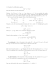### List of available CDs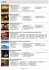### south east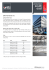### + m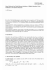### Yancy y Amigos Pequeña Fiesta de Alabanza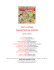### contractive multi-valued mappings on alpha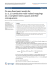### Classification of endomorphisms of some Lie algebroids up to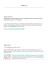### Let`s Cook Multi Chef 162246.book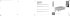### 03_studi sul libro per ragazzi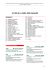### Abstract Algebra